Thevenin Theorem This theorem is very conceptual. If we think deeply about an electrical circuit , we can visualize the statements mad...

## Thevenin Theorem

This theorem is very conceptual. If we think deeply about an electrical circuit, we can visualize the statements made in Thevenin theorem. Suppose we have to calculate the current through any particular branch in a circuit. This branch is connected with rest of the circuits at its two terminal. Due to active sources in the circuit, there is one electric potential difference between the points where the said branch is connected. The current through the said branch is caused by this electric potential difference that appears across the terminals. So rest of the circuit can be considered as a single voltage source, that's voltage is nothing but the open circuit voltage between the terminals where the said branch was connected and the internal resistance of the source is nothing but the equivalent resistance of the circuit looking back into the terminals where, the branch was connected. So the Thevenin theorem can be stated as follows,

An active bilateral linear network containing energy sources (generators) and impedances can be replaced by an equivalent circuit containing a voltage source (ETh or VTh) in series with an impedance (ZTh), where the ETh or VTh is the open circuit voltage between terminals of the network and ZTh is the impedance measured between the terminals of this network with all energy sources eliminated (not eliminating their impedances).

In other words
1.When a particular branch is removed from a circuit, the open circuit voltage appears across the terminals of the circuit, is Thevenin equivalent voltage and,
2.The equivalent resistance of the circuit network looking back into the terminals, is Thevenin equivalent resistance.
3.If we replace the rest of the circuit network by a single voltage source, then the voltage of the source would be Thevenin equivalent voltage and internal resistance of the voltage source would be Thevenin equivalent resistance which would be connected in series with the source as shown in the figure below.

The load current is calculated as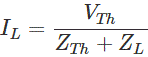Where, ZL is the Load impedance and ZTh is the internal impedance of the circuit as viewed back into the open circuited network from terminal A & B with all voltage sources replaced by their internal impedances (if any) current sources with infinite impedance.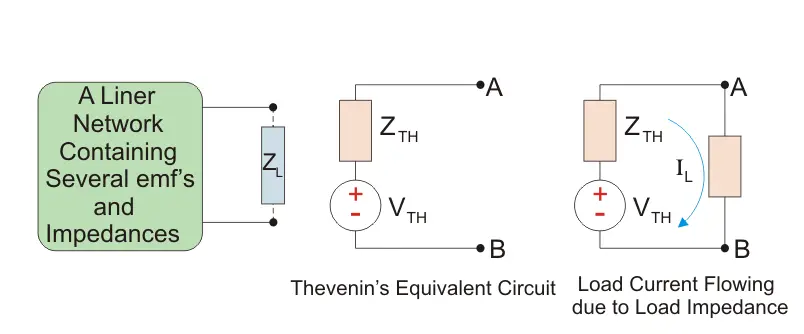Some Important Terms to be kept in mind
1.Bilateral network is a network which does not change its characteristics due to change the direction of its voltage and current sources. Bilateral network elements are R, L, and C.
2.Linear Network is a network in which its parameters (like resistor, capacitor and inductor) never change their magnitude with respect to the variation of current or voltage or both.
3.Unilateral network always change its characteristics with respect to the change of direction of voltage and current. Example: vacuum diode, silicon diode, crystal detectors etc

To make Thevenin theorem easy to understand, we have shown the circuit below,

Here two resistors R1 and R2 are connected in series and this series combination is connected across one voltage source of emf E with internal resistance Ri as shown. One resistive branch of RL is connected across the resistance R2 as shown. Now we have to calculate the current through RL.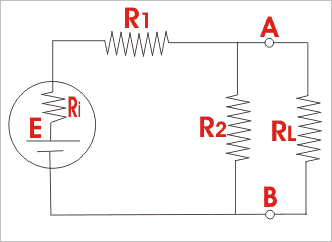First, we have to remove the resistor RL from the terminals A and B.

Second, we have to calculate the open circuit voltage or Thevenin equivalent voltage VT across the terminals A and B.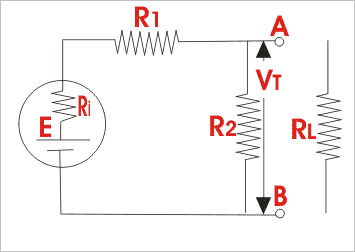The current through resistance R2,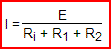Hence voltage appears across the terminals A and B i.e.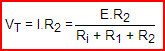Third, for applying Thevenin theorem, we have to determine the Thevenin equivalent electrical resistance of the circuit, and for that; first we have to replace the voltage source from the circuit, leaving behind only its internal resistance Ri. Now view the circuit inwards from the open terminals A and B. It is found the circuits now consist of two parallel paths - one consisting of resistance R2 only and the other consisting of resistance R1 and Ri in series.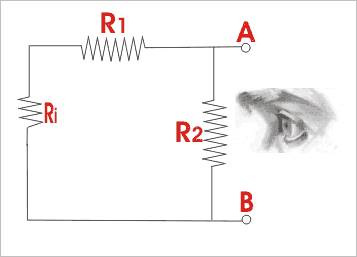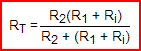Thus the Thevenin equivalent resistance RT is viewed from the open terminals A and B is given as. As per Thevenin theorem, when resistance RL is connected across terminals A and B, the network behaves as a source of voltage VT and internal resistance RT and this is called Thevenin equivalent circuit. The current through RL is given as,

## Thevenin Equivalent Circuit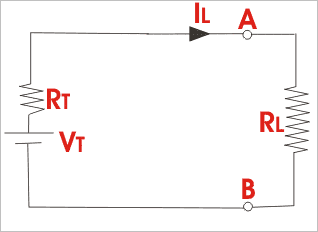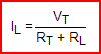### Application of Thevenin’s Theorem in DC Network

If you apply Thevenin’s theorem in DC system, then ZTh can be considered as RTh. Whatever may be the circuit, we have to make that one as per the circuit shown below.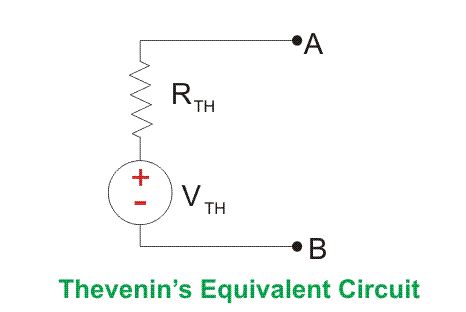Load resistance RL is inserted to find out the Load current, following the figure below.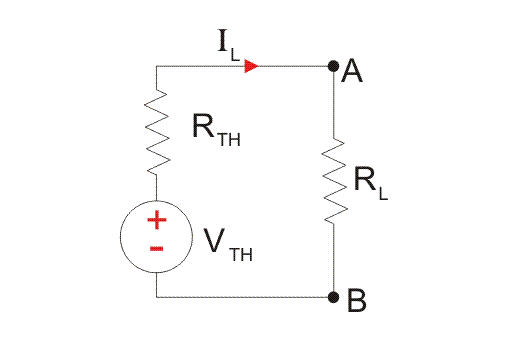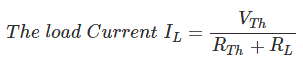Procedure to Solve a Problem by Applying Thevenin’s Theorem

Some important steps are to be followed carefully.
Step 1: Draw the circuit by removing load resistance, shortening voltage sources and opening the current sources from the circuit. Name the Load terminals with A & B.
Step 2: View back into the open circuited network i.e. from the open terminal A & B. Calculate equivalent resistance of the circuit, i.e. RTh.
Step 3: Draw the circuit as previous but keeping the Load Resistance removed from A & B terminal.
Step 4: Find the individual Loops. Apply KVL (Kirchhoff’s Voltage Law) and find out loop current.
Step 5: Start journey from terminal A to B by choosing any path of branches. Calculate total Voltage that you have faced during journey. This voltage is VTh.
Step 6: Draw the Thevenin’s equivalent circuit with the value of calculate RTh and VTh. Connect RL across AB terminal. Again apply KVL to find out the load current IL directly put the value of VTh, RTh and RL in the formula
•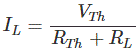Some important conventions to solve a circuit by applying Thevenin’s Theorem
When you travel along a branch against the direction of the current flowing, take the voltage for the resistance R as positive voltage drop or + IR.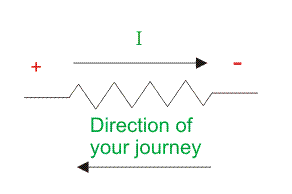When you travel along a branch in the direction of the current flowing, take the voltage drop for the resistance R as negative voltage drop or – IR.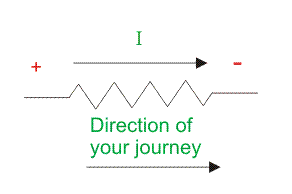When you apply KVL consider the direction of current in a loop clock wise always whatever may be the sign of the current or actual direction of the actual current.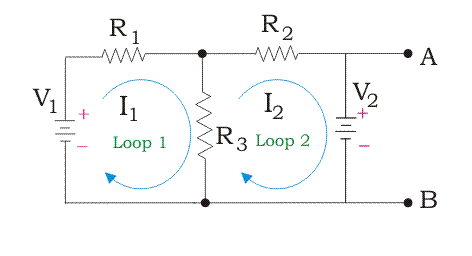Get familiar with Thevenin’s Theorem by applying on a Circuit to find out Load Current
Suppose the electrical circuit is like that,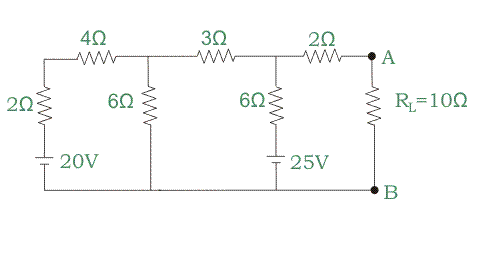Now follow the steps one by one.
Step 1: Draw the circuit by removing load resistance, shortening voltage sources and opening the current sources (if any) from the circuit. Name the Load terminals with A & B.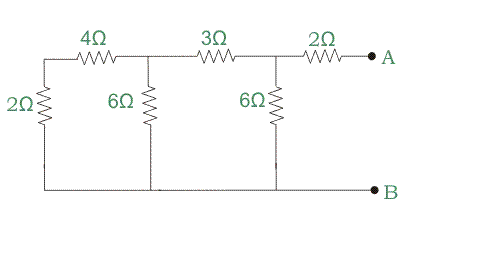Step 2: View back into the open circuited network i.e. from the open terminal A & B. Calculate equivalent resistance of the circuit, i.e. RTh.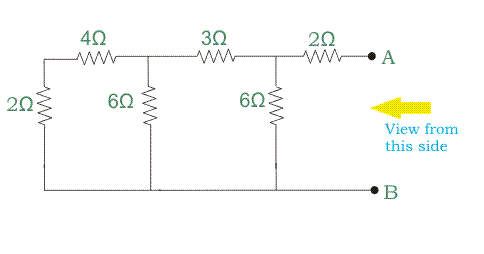Now calculate the internal resistance of the network.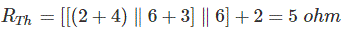You got the value of RTh = 5ohm. Of the Thevenin’s equivalent circuit.
So go for the next steps to find out the value of VTh.
Step 3: Draw the circuit as previous but keeping the Load Resistance removed from A & B terminal.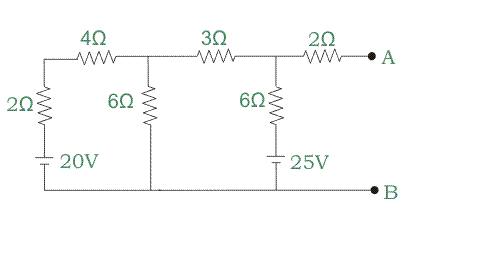Step 4: Find the individual Loops. Apply KVL (Kirchhoff’s Voltage Law) and find out loop current.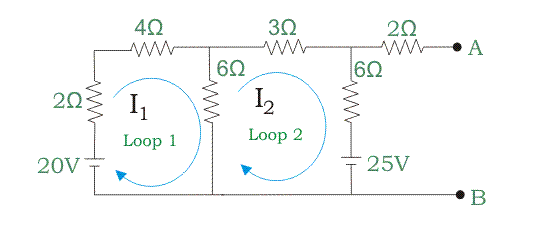You got two individual two loops from the circuit. Mark the loops with clock wise arrow as the direction of the current flowing.
Now start to apply KVL in the first loop.[as you are in the loop 1, consider I1 > I2, through 6 ohm resistor (I1 – I2) current will flow in]By applying KVL in the second loop, we get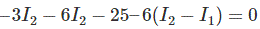[As you are in the loop 2, consider I2 > I1, through 6 ohm resistor (I2 – I1) current will flow in]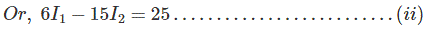By solving two equations we get the value of I1 = 1.041A and I2 = 1.25 A.
So the actual direction of the currents is marked in the figure below.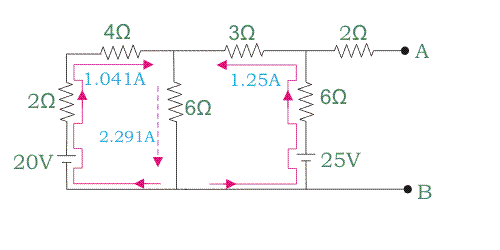Step 5: Start journey from terminal A to B by choosing any path of branches. Calculate total Voltage that you have faced during journey. This voltage is VTh.
Assume this VTh connected across A and B terminal.
From terminal A start your journey along any branch to reach terminal B.
Let us start journey as per marked path by Red Color.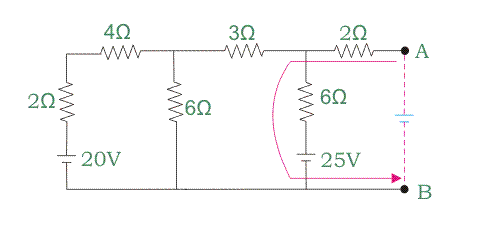Now by applying KVL again, we can write that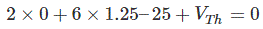[There is no current through 2 ohm resistor just connected to terminal A]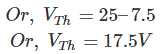So Thevenin’s Voltage VTh is 17.5V.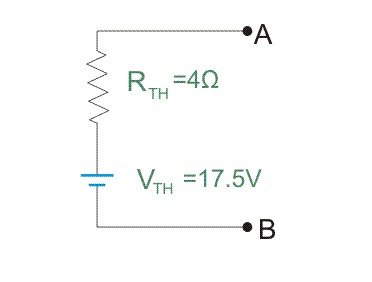You can verify this value of VTh choosing another path in the circuit from terminal A to B. Let us choose another path as per drawing below.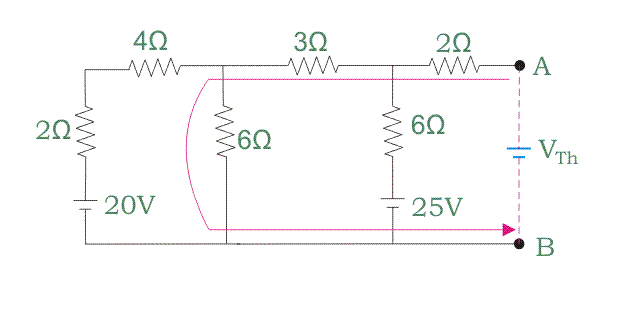Now applying KVL, we get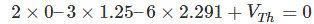[there is no current through 2ohm resistor just connected to terminal A]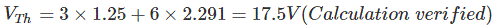Step 6: Draw the Thevenin’s equivalent circuit with the value of calculate RTh and VTh. Connect RL across AB terminal. Again apply KVL to find out the load current IL or directly put the value of VTh, RTh and RL in the formula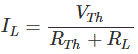Now connect RL = 10 ohm across A and B terminal. Again apply KVL here and get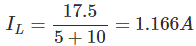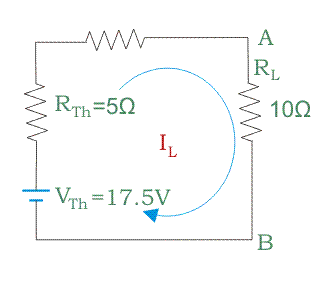## Application of Thevenin’s Theorem in AC System

To calculate VTh and ZTh we have to follow the steps which are followed in DC system to solve a problem. But one thing extra i.e. phase angle consideration as it AC system. Let’s start to solve a problem and get familiar with the steps again. Suppose the circuit is like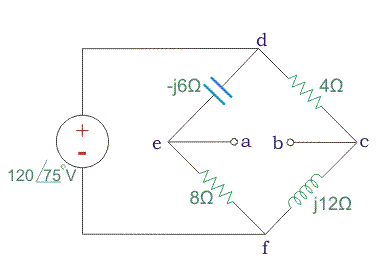Here terminal A and B are load terminals. Step 1: Draw the circuit by removing load resistance, shortening voltage sources and opening the current sources from the circuit.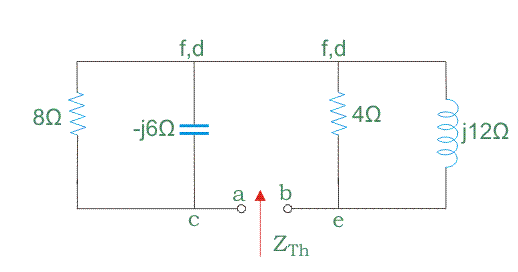Step 2: View back into the open circuited network i.e. from the open terminal A & B. Calculate equivalent resistance of the circuit, i.e ZTh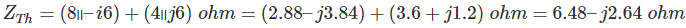Step 3: Draw the circuit as previous but keeping the Load Resistance removed from A & B terminal.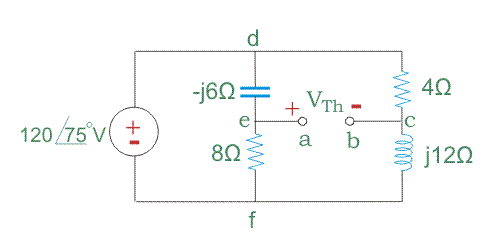Step 4: Find the individual Loops. Apply KVL (Kirchhoff’s Voltage Law) and find out loop current.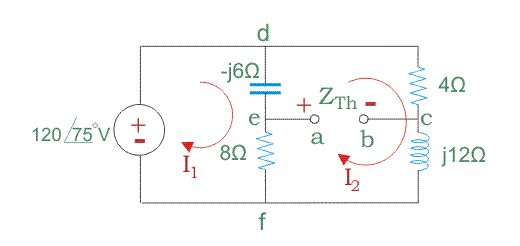For the loop 1,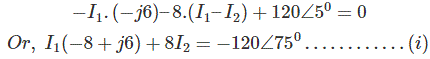For the loop 2,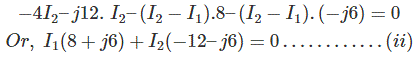Solving them we get,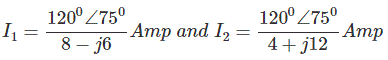Step 5: Start journey from terminal A to B by choosing any path of branches. Calculate total Voltage that you have faced during journey. This voltage is VTh. Choose a path as per red marked way on the figure below.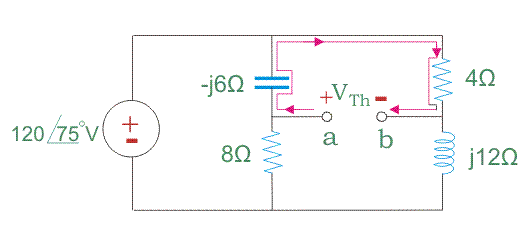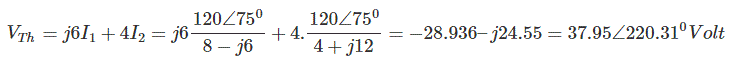A special Problem considering mutual induction in the circuit Without any mutual induction application of Thevenin’s theorem is easily applicable to find out VTh and ZTh. But when there the effect of mutual induction in the circuit then a special approach to be considered along with the general steps. This type of circuit is given below.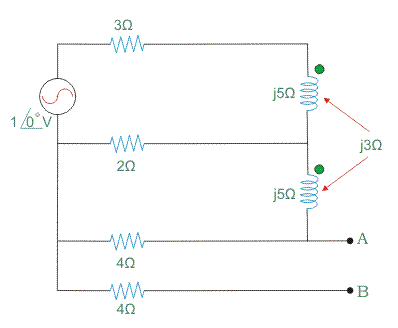First draw this circuit as per presence of mutual inductance.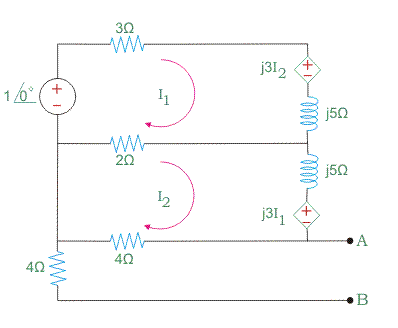Now follow the steps one by one. But a problem will arise to find out ZTh. But short cut and easy method is that connect a voltage source of 1 volt across A & B terminal and remove the volage sources from the circuit. Now appy KVL in each loop. Hence calculate the value Of I3 only.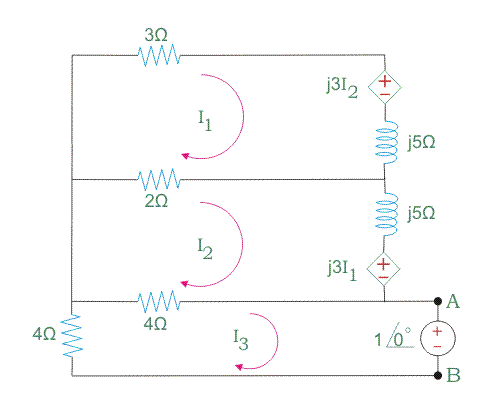Now the value of ZTh = -1∠0o/I3 ohm. But to find out VTh only you need to calculate the value of I3 from the given circuit below.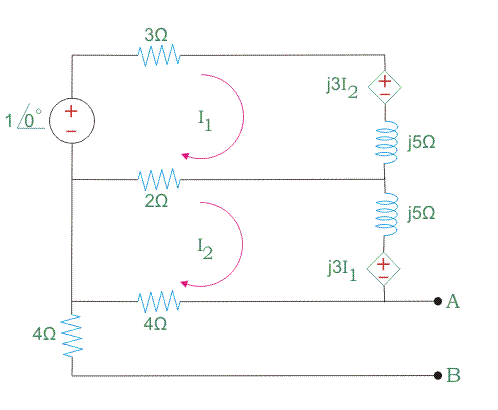Now calculate the value of I2. VTh = I2.4 volt. So you get the data of the equvalent circuit of Thevenin.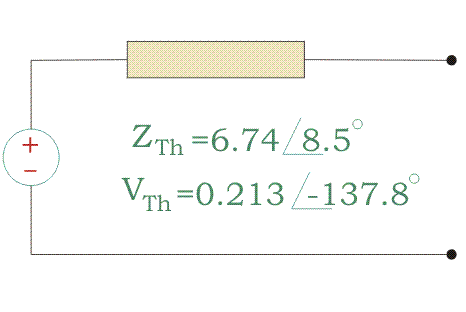Name

BASIC ELECTRICAL,12,BATTERIES,4,CIRCUIT THEORIES,9,CONTROL SYSTEMS,3,DC MOTOR,1,DIGITAL ELECTRONICS,1,DISTRIBUTED GENERATION,2,DISTRIBUTION,6,ELECTRICAL DRIVES,1,ELECTRICAL LAWS,8,ELECTRONICS DEVICES,2,General,7,GENERATION,3,GENERATOR,1,HIGH VOLTAGE,4,ILLUMINATION,1,INDUCTION MOTOR,7,MATERIALS,1,MEASUREMENT,1,MOTOR,1,POWER ELECTRONICS,2,PROJECTS ON INDUCTION MOTOR,1,PROTECTION,1,SMART GRID,3,SWITCHGEAR,4,SYNCHRONOUS MOTOR,1,TRANSFORMER,6,TRANSMISSION,4,
ltr
item
Electrical for Us: Thevenin Theorem and Thevenin Equivalent Voltage and Resistance
Thevenin Theorem and Thevenin Equivalent Voltage and Resistance
https://3.bp.blogspot.com/-OQWXv4b6myI/WD8TlkO6wXI/AAAAAAAAAb4/texn9BaVbYcJ7Z3qOGTMsS6J9lyEjYW7QCPcB/s320/thevenins_%2526_nortons_theorem.jpg
https://3.bp.blogspot.com/-OQWXv4b6myI/WD8TlkO6wXI/AAAAAAAAAb4/texn9BaVbYcJ7Z3qOGTMsS6J9lyEjYW7QCPcB/s72-c/thevenins_%2526_nortons_theorem.jpg
Electrical for Us
http://www.sanjaysah.com.np/2016/11/thevenin-theorem-and-thevenin.html
http://www.sanjaysah.com.np/
http://www.sanjaysah.com.np/
http://www.sanjaysah.com.np/2016/11/thevenin-theorem-and-thevenin.html
true
3851448774078769448
UTF-8